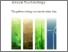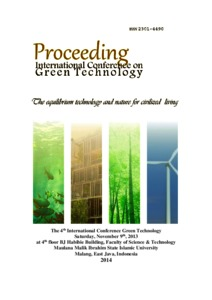# Discretization reaction-diffusion models with finite difference method

Pagalay, Usman and Efendi, Ernawati and Kusumastuti, Ari (2013) Discretization reaction-diffusion models with finite difference method. Presented at International Conference on Green Technology, 09 November 2013, UIN Maulana Malik Ibrahim Malang, Indonesia. (Unpublished)Preview
Text (full text)
1816.pdf

## Abstract

Discretization model is a continuous model transformation procedure to model discrete. Discretization is done using advanced finite difference method, by analogy differential equations using limit rules, with different equations using the different between discrete time points. The model used in this paper is a model of reaction-diffusion (Turing) that represents the diffusion of fluid in the cells that cause the cells to move. Finite difference method is a numerical method that can be used to solve partial differential equations. Methods used explicit finite difference scheme developed for the time difference and central difference for the space to complete the reactiondiffusion equation (Turing). Based on the numerical solution obtained then the amount of domain growth does not affect the stability of reaction-diffusion models (Turing).

Item Type: Conference (Paper) Keywords Discretization, reaction-diffusion (Turing) model, forward finite differences method, continuous model, discrete model. 01 MATHEMATICAL SCIENCES > 0102 Applied Mathematics > 010202 Biological Mathematics Faculty of Mathematics and Sciences > Department of Biology Usman Pagalay 27 Jul 2017 04:43View Item